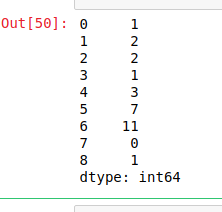# Python | Pandas Series.combine()

Python is a great language for doing data analysis, primarily because of the fantastic ecosystem of data-centric Python packages. Pandas is one of those packages and makes importing and analyzing data much easier.

Pandas `Series.combine()` is a series mathematical operation method. This is used to combine two series into one. The shape of output series is same as the caller series. The elements are decided by a function passed as parameter to `combine()` method. The shape of both series has to be same otherwise it will throw an error.

Syntax: Series.combine(other, func, fill_value=nan)

Parameters:
other: other series or list type to be combined with caller series
func: Function passed as parameter which will decide from which series the element should be put at that index
fill_value: integer value of level in case of multi index

Return: Combined series with same shape as caller series

Example #1:

In this example, two lists are made and converted into pandas series using .Series() method. A function is made using lambda which checks which values is smaller in both series and returns whichever is the smaller.

 `# importing pandas module ` `import` `pandas as pd ` ` `  `# creating first series ` `first ``=``[``1``, ``2``, ``5``, ``6``, ``3``, ``7``, ``11``, ``0``, ``4``] ` ` `  `# creating second series ` `second ``=``[``5``, ``3``, ``2``, ``1``, ``3``, ``9``, ``21``, ``3``, ``1``] ` ` `  `# making series ` `first ``=` `pd.Series(first) ` ` `  `# making seriesa ` `second ``=` `pd.Series(second) ` ` `  `# calling .combine() method ` `result ``=` `first.combine(second, (``lambda` `x1, x2: x1 ``if` `x1 < x2 ``else` `x2)) ` ` `  `# display ` `result `

Output:
As shown in the output image, the returned series is having smaller values from both series.Example #2:

In this example, Null values are passed too using `Numpy.nan` method. Since series contains null values, 5 is passed to fill_value parameter to replace null values by 5. A lambda function is passed which will compare values in both series and will return the greater one.

 `# importing pandas module ` `import` `pandas as pd ` ` `  `# importing numpy module ` `import` `numpy as np ` ` `  `# creating first series ` `first ``=``[``1``, ``2``, np.nan, ``5``, ``6``, ``3``, np.nan, ``7``, ``11``, ``0``, ``4``, ``8``] ` ` `  `# creating second series ` `second ``=``[``5``, ``3``, ``2``, np.nan, ``1``, ``3``, ``9``, ``21``, ``3``, np.nan, ``1``, np.nan] ` ` `  `# making series ` `first ``=` `pd.Series(first) ` ` `  `# making seriesa ` `second ``=` `pd.Series(second) ` ` `  `# calling .combine() method ` `result ``=` `first.combine(second, func ``=``(``lambda` `x1, x2: x1 ``if` `x1 > x2 ``else` `x2), fill_value ``=` `5``) ` ` `  `# display ` `result `

Output:
As shown in the output, the NaN values in the series were replaced by 5 before combining the series.

```
0      5.0
1      3.0
2      2.0
3      5.0
4      6.0
5      3.0
6      9.0
7     21.0
8     11.0
9      5.0
10     4.0
11     8.0
dtype: float64```

Whether you're preparing for your first job interview or aiming to upskill in this ever-evolving tech landscape, GeeksforGeeks Courses are your key to success. We provide top-quality content at affordable prices, all geared towards accelerating your growth in a time-bound manner. Join the millions we've already empowered, and we're here to do the same for you. Don't miss out - check it out now!

Previous
Next